# Orthomodular lattice

(diff) ← Older revision | Latest revision (diff) | Newer revision → (diff)
Jump to: navigation, search

A lattice with a zero

and a one

in which for any elementthere is an orthocomplement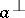, i.e. an element such that

1)2)and such that the orthomodular law:

3)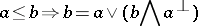is satisfied.

In an orthomodular lattice one studies distributivity, perspectivity, irreducibility, modularity of pairs, properties of the centre and of ideals, the commutator, solvability, and applications in the logic of quantum mechanics (see , ).

Ifis an arbitrary von Neumann algebra, then the set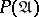of all its projections is a complete orthomodular lattice. In these conditions, ifis a factor, then a dimension function can be defined on the set. Dependent on the set of values of this function, the factors are divided into the types,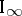,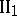,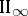,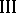(the Murray–von Neumann classification, see ). It has been established that lattices of projections of factors of the typeandare continuous geometries, i.e. complete complemented modular lattices (cf. Lattice with complements; Modular lattice; Complete lattice) satisfying the following two continuity axioms:

1)for any directed set of indicesand any set of elements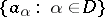such that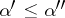implies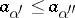;

2) the condition dual to 1).

The problem of constructing an abstract dimension theory within the framework of such a class of lattices, which would also include, apart from modular lattices of projections of factors of the types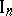and, non-modular lattices of projections of factors of the remaining types, has arisen. The existence of a dimension function of a complete orthomodular lattice with an equivalence relation which satisfies certain supplementary conditions has been proved. This class of lattices includes both lattices of projections of factors and continuous geometries.

Orthomodular lattices, which are a natural generalization of lattices of projections of factors, also constitute an essentially broader class, in that many properties of lattices of projections are not valid for arbitrary orthomodular lattices. In the same way as continuous geometries are coordinatized by regular rings (see ), orthomodular lattices can be coordinatized by Baer-semi-groups. If a complete orthomodular lattice is modular, it is continuous (see ). There exists an orthocomplemented modular lattice whose completion by sections is not orthomodular (whereas the completion by sections of a semi-modular orthocomplemented lattice is semi-modular and the lattice of projections of a von Neumann algebra is semi-modular).

How to Cite This Entry:
Orthomodular lattice. Encyclopedia of Mathematics. URL: http://encyclopediaofmath.org/index.php?title=Orthomodular_lattice&oldid=12374
This article was adapted from an original article by T.S. Fofanova (originator), which appeared in Encyclopedia of Mathematics - ISBN 1402006098. See original article